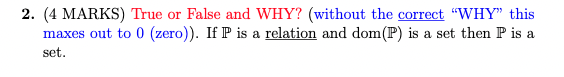# Question 2. (4 MARKS) True or False and WHY? (without the correct "WHY" this maxes out to 0 (zero)). If $$\mathbb{P}$$ is a relation and $$\operatorname{dom}(\mathbb{P})$$ is a set then $$\mathbb{P}$$ is a set.Transcribed Image Text: 2. (4 MARKS) True or False and WHY? (without the correct "WHY" this maxes out to 0 (zero)). If $$\mathbb{P}$$ is a relation and $$\operatorname{dom}(\mathbb{P})$$ is a set then $$\mathbb{P}$$ is a set.
Transcribed Image Text: 2. (4 MARKS) True or False and WHY? (without the correct "WHY" this maxes out to 0 (zero)). If $$\mathbb{P}$$ is a relation and $$\operatorname{dom}(\mathbb{P})$$ is a set then $$\mathbb{P}$$ is a set.